# Calculus 3 : Fundamental Theorem of Line Integrals

## Example Questions

### Example Question #1 : Fundamental Theorem Of Line Integrals

Evaluate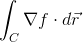, where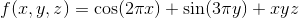, andis any path that starts at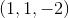, and ends at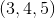.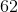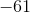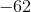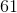Explanation:

Since there isn't a specific path we need to take, we just evaluate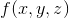at the end points.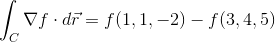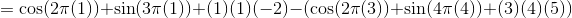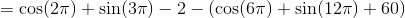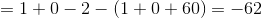### All Calculus 3 Resources